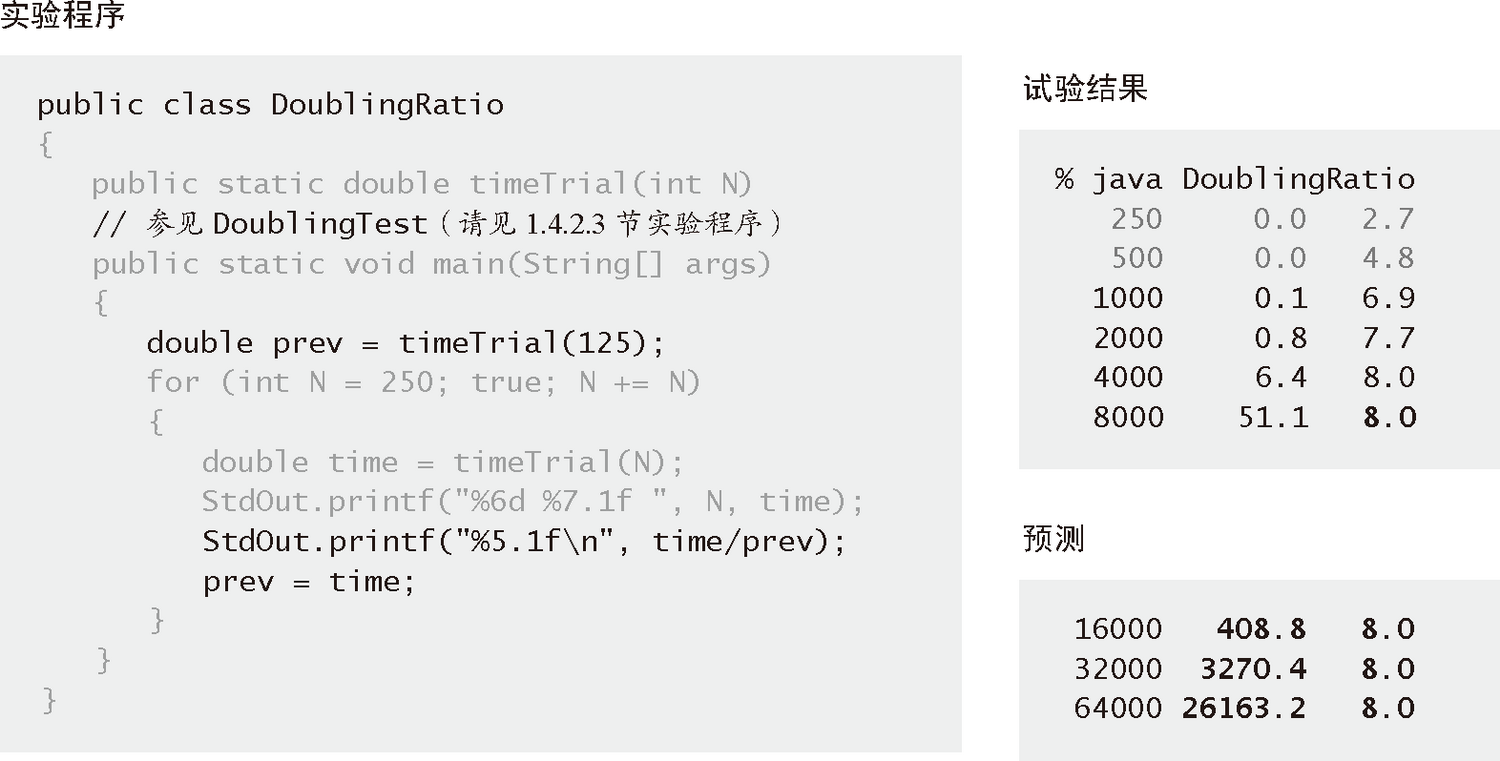# 算法（4th ed）(170)：基础——算法分析 6.6(倍率实验）

• 开发一个输入生成器来产生实际情况下的各种可能的输入（例如 DoublingTest 中的 timeTrial() 方法能够生成随机整数）。
• 运行下方的 DoublingRatio 程序，它是 DoublingTest 的修改版本，能够计算每次实验和上一次的运行时间的比值。
• 反复运行直到该比值趋近于极限 $2^{{\rm b}}$

• 它们的运行时间的增长数量级约为 $N^{{\rm b}}$
• 要预测一个程序的运行时间，将上次观察得到的运行时间乘以 $2^{{\rm b}}$ 并将 $N$ 加倍，如此反复。如果你希望预测的输入规模不是 $N$ 乘以 2 的幂，可以相应地调整这个比例（请见练习 1.4.9）。\begin{aligned}{\rm T}(2N)/{\rm T}(N)&=a(2N)^b\lg(2N)/aN^b\lg N\&=2^b(1+\lg2/\lg N)\&\sim2^b\end{aligned}